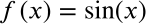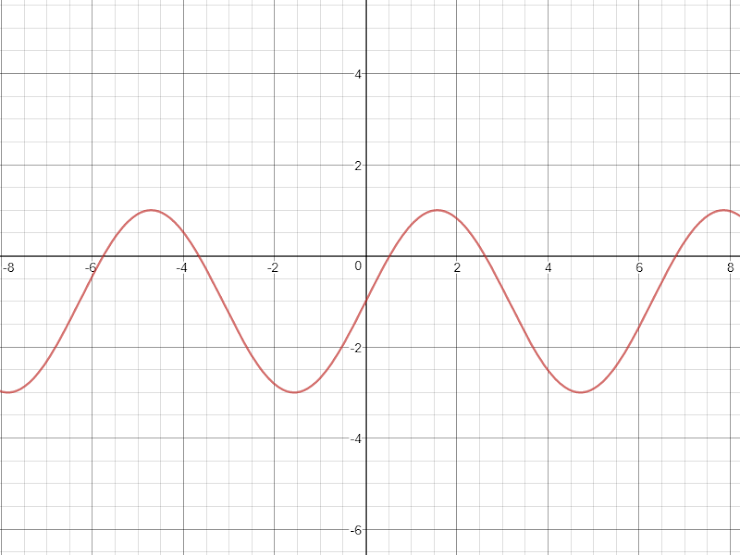Exit Ticket for Day 5 of Trig
Please select your name from this list: *
1. Which of the following is the graph of the equation shown below?2. Which of the following is the graph of f(x)=3cos(x) ? (The graph will be the BLACK curve... the RED curve is the graph of cos(x), just for comparison.)
3. The following is a graph of f(x)=asin(x)+d. What are the values of a and d?4. For a person at rest, the velocity v (in liters per second) of air flow during a respiratory cycle (that is, the time from the beginning of one breath to the beginning of the next breath) is given by the given equation. Here, t is in "seconds", v>0 means that you are inhaling and v<0 means that you are exhaling. FIND THE TIME FOR ONE FULL RESPIRATORY CYCLE.This form was created inside of Evansville Vanderburgh School Corporation. Report Abuse - Terms of Service